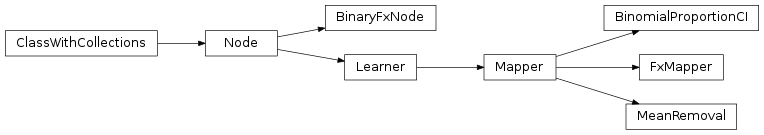# mvpa2.mappers.fx¶

Transform data by applying a function along samples or feature axis.Functions

 `absolute_features`() Returns a mapper that converts features into absolute values. `argsort`(seq[, reverse]) Return indices to get sequence sorted `array_whereequal`(a, x) Reliable comparison for `numpy.ndarray` `borrowdoc`(cls[, methodname]) Return a decorator to borrow docstring from another `cls`.`methodname` `expand_contraint_spec`(spec) Helper to translate literal contraint specs into functional ones `max_of_abs`(x) Max of absolute values along the 2nd axis `maxofabs_sample`() Returns a mapper that finds max of absolute values of all samples. `mean_feature`([attrfx]) Returns a mapper that computes the mean feature of a dataset. `mean_group_feature`(attrs[, attrfx]) Returns a mapper that computes the mean features of unique feature groups. `mean_group_sample`(attrs[, attrfx]) Returns a mapper that computes the mean samples of unique sample groups. `mean_sample`([attrfx]) Returns a mapper that computes the mean sample of a dataset. `merge2first`(attrs) Compress a sequence by discard all but the first element `subtract_mean`(x) Subtract mean across first axis `subtract_mean_feature`([attrfx]) Subtract mean of features across samples. `sum_of_abs`(x) Sum of absolute values along the 2nd axis `sum_sample`([attrfx]) Returns a mapper that computes the sum sample of a dataset. `sumofabs_sample`() Returns a mapper that returns the sum of absolute values of all samples.

Classes

 `AltConstraints`(\*constraints) Logical OR for constraints. `BinaryFxNode`(fx, space, \*\*kwargs) Extract a dataset attribute and call a function with it and the samples. `BinomialProportionCI`(\*\*kwargs) Compute binomial proportion confidence intervals `Constraint` Base class for input value conversion/validation. `Constraints`(\*constraints) Logical AND for constraints. `Dataset`(samples[, sa, fa, a]) Generic storage class for datasets with multiple attributes. `EnsureBool` Ensure that an input is a bool. `EnsureChoice`(\*values) Ensure an input is element of a set of possible values `EnsureDType`(dtype) Ensure that an input (or several inputs) are of a particular data type. `EnsureFloat`() Ensure that an input (or several inputs) are of a data type ‘float’. `EnsureInt`() Ensure that an input (or several inputs) are of a data type ‘int’. `EnsureListOf`(dtype) Ensure that an input is a list of a particular data type `EnsureNone` Ensure an input is of value `None` `EnsureRange`([min, max]) Ensure an input is within a particular range `EnsureStr` Ensure an input is a string. `EnsureTupleOf`(dtype) Ensure that an input is a tuple of a particular data type `FxMapper`(axis, fx[, fxargs, uattrs, attrfx, ...]) Apply a custom transformation to (groups of) samples or features. `Mapper`(\*\*kwargs) Basic mapper interface definition. `MeanRemoval`([in_place]) Subtract sample mean from features. `Node`([space, pass_attr, postproc]) Common processing object. `Parameter`(default[, constraints, ro, index, ...]) This class shall serve as a representation of a parameter.